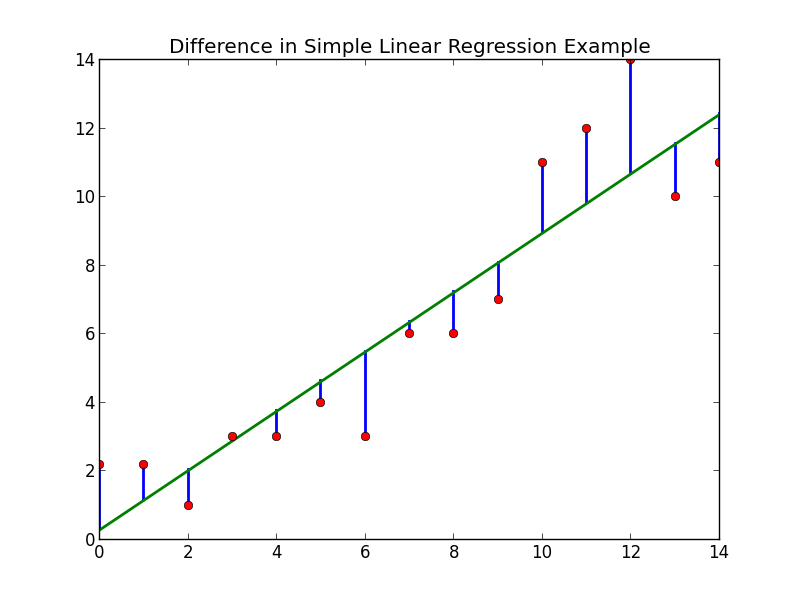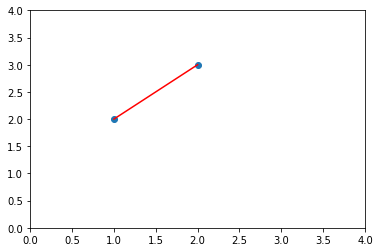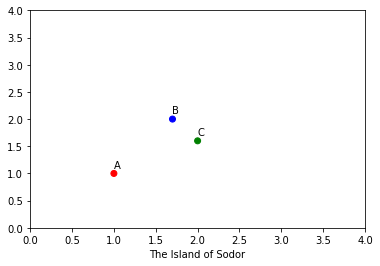### 投影问题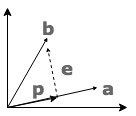$\hat{\vec{\theta}}$ 即是我们的误差表示的最优解。些时投影为 $Proj = X \cdot \hat{\vec{\theta}} = X \cdot (X^T X)^{-1} X ^ T \vec{y}$, 而 $P = X \cdot (X^T X)^{-1} X ^ T$ 为投影矩阵。

### 多多岛的解决方案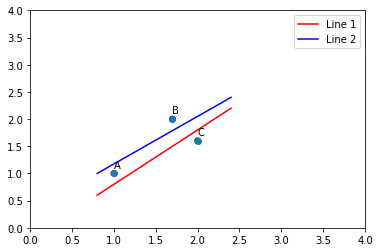X = [1 1 1; 1 1.7 2; 1 2 1.6]
y = [1;1;-1]
thetas = inv(X.'*X)*X.'*y


### 代码实现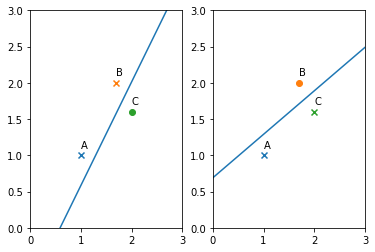import numpy as np

def get_LSE_weights(yLabels):
''' 最小二乘法 '''
y = np.mat(yLabels).T
X = np.mat([[1, 1, 1], [1, 1.7, 2], [1, 2, 1.6]])
return (X.T * X).I * X.T * y

def plotLine(ax, weights):
x_1 = np.arange(0, 3.5, 0.1)
x_2 = (-weights - weights * x_1) / weights # x2 = (-w0 - w1*x1)/w2
ax.plot(x_1, np.squeeze(np.asarray(x_2)))

def plotIsland(ax, y):
t = ['A', 'B', 'C']
ax.set_xlim([0, 3])
ax.set_ylim([0, 3])
x1 = [1, 1.7, 2]
x2 = [1, 2, 1.6]

for i in range(3):
m = 'x'
if y[i] == -1:
m = 'o'
ax.scatter(x1[i], x2[i], marker=m)
ax.annotate(t[i], (x1[i], x2[i] + 0.1))

fig, (ax1, ax2) = plt.subplots(1, 2)

yLabels1 = [1, 1, -1]
plotIsland(ax1, yLabels1)
yLabels2 = [1, -1, 1]
plotIsland(ax2, yLabels2)

weights = get_LSE_weights(yLabels1)
plotLine(ax1, weights)

weights = get_LSE_weights(yLabels2)
plotLine(ax2, weights)

plt.show()